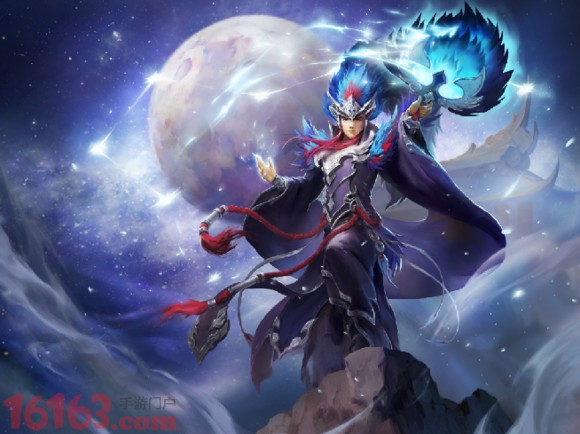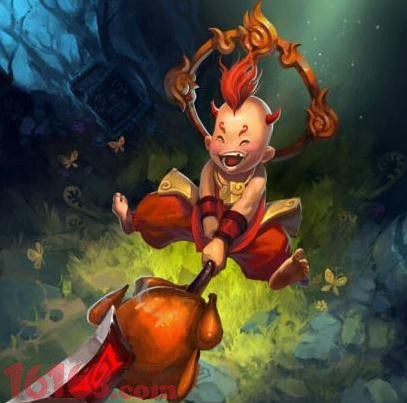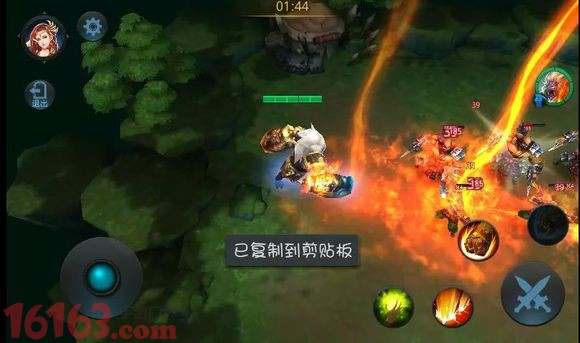# 乱斗西游2昴日星官玩法实测 数据全面分析

乱斗西游2昴日星官是新的英雄，不知道有没有小伙伴使用过了，估计有很多小伙伴都想知道昂日星官在各个玩法中表现如何，下面就来看看乱斗西游2昴日星官玩法实测，数据全面分析哦！技能数据分析：

一、飞星：AP加成：59÷314=0.19

二、拱辰：AP加成：113÷314=0.36

三、昴日：AP加成：88÷314=0.28

当法强314时【物强日后太低，忽略不计】

不暴击不附加不状态纯输出伤害：【不算入拱辰昴日结合伤害等】

164+268+208=644

设定星雨三件套加江湖夜雨，仙缘暴击五星满，暴击伤害加成：8×3+3+6+5+150=188%

那么按照技能132的顺序放，设定1.2必定暴击，3由1暴击而暴击：

加了状态的伤害：308+503+391+196+392=1790

此伤害为56篝火一轮输出纯伤害【不算普攻】

我们再来算算同为纯属出暴击英雄红孩儿的数据AP加成：

一、105÷314=0.33

二、105÷134=0.33

三、105÷314=0.33

红孩儿三个技能AP加成相同，略高于星官

当法强314时【物强日后太低，忽略不计】

不暴击不附加不状态纯输出伤害：195+225+325×3=1395

设定星雨三件套加江湖夜雨，仙缘暴击五星满，暴击伤害加成：8×3+3+6+5+150=188%

设定技能23必定暴击伤害：195+423+1883=2451

AP加成：

一、105÷314=0.33

二、105÷134=0.33

三、105÷314=0.33

红孩儿三个技能AP加成相同，略高于星官

当法强314时【物强日后太低，忽略不计】

不暴击不附加不状态纯输出伤害：195+225+325×3=1395

设定星雨三件套加江湖夜雨，仙缘暴击五星满，暴击伤害加成：8×3+3+6+5+150=188%

设定技能23必定暴击伤害：195+423+1883=2451

然后我们再看看热门的禺狨王

一、62÷392=0.16

二、81÷392=0.20

三、116÷392=0.30

看下面一张图由图知当法强为392时附加伤害：

引爆：185 灼烧：39

自动时大概技能13间隔1秒，所以总灼烧9秒

当总强度为314时：

不暴击不附加不状态纯输出伤害：【不加灼烧引爆等】

114+123+184=423

设定星雨三件套加江湖夜雨，仙缘暴击五星满，暴击伤害加成：8×3+3+6+5+150=188%

设定技能23必定暴击，伤害：

114+231+346+120+279=1090【总强度定为314，与前两个相同】

最后我们对比一下数据：

星1790，红2451，羽1090【不加普攻】。从这个数据可以看出星官在打56篝火时远远不如红孩子禺狨王毕竟是个群攻嘛，比不过前两个。

从红孩子不太苛刻的暴击要求可以得出：

排行、56篝火、修罗方面星官确实不如孩子，虽然羽绒单伤低了点，但群伤可怕。

以上就是乱斗西游2昴日星官玩法实测，希望对大家有所帮助！